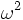# Supercharacter theories for alternating group:A4

## Contents

View supercharacter theories for particular groups | View other specific information about alternating group:A4

This page discusses the various possible supercharacter theories for alternating group:A4. Thus, it builds on a thorough understanding of the element structure of alternating group:A4, subgroup structure of alternating group:A4, and linear representation theory of alternating group:A4.

We describe the group$A_4$ as the alternating group on$\{ 1,2,3,4 \}$, and elements of the group are described by means of their cycle decompositions.

## Character table

Below, the character table of$A_4$ is given. This table is crucial for understanding the possible supercharacter theories.

Let$\omega$ be a primitive cube root of unity. The character table over a splitting field is as follows:

Representation/conjugacy class representative$()$ (size 1)$(1,2)(3,4)$ (size 3)$(1,2,3)$ (size 4)$(1,3,2)$ (size 4)
trivial representation 1 1 1 1
first nontrivial one-dimensional representation 1 1$\omega$$\omega^2$
second nontrivial one-dimensional representation 1 1$\omega^2$$\omega$
three-dimensional irreducible representation 3 -1 0 0

## Supercharacter theories

Note that for each of the supercharacter tables presented, the supercharacter is the smallest positive integer linear combination of the characters in the block that takes constant values on each superconjugacy class.

### Summary

Quick description of supercharacter theory Number of such supercharacter theories under automorphism group action Number of blocks of conjugacy classes = number of blocks of irreducible representations Block sizes for conjugacy classses (in number of conjugacy class terms) (should add up to 4, the total number of conjugacy classes) Block sizes for conjugacy classes (in number of elements terms) (should add up to 12, the order of the group) Block sizes for irreducible representations (in number of representations terms) (should add up to 4, the total number of conjugacy classes) Block sizes for irreducible representations (in sum of squares of degrees terms) (should add up to 12, the order of the group)
ordinary character theory 1 4 1,1,1,1 1,3,4,4 1,1,1,1 1,1,1,9
all non-identity elements form one block 1 2 1,3 1,11 1,3 1,11
corresponds to normal series with V4 in A4 as the middle subgroup 1 3 1,1,2 1,3,8 1,1,2 1,2,9
Total (3 rows) 3 (equals total number of supercharacter theories)